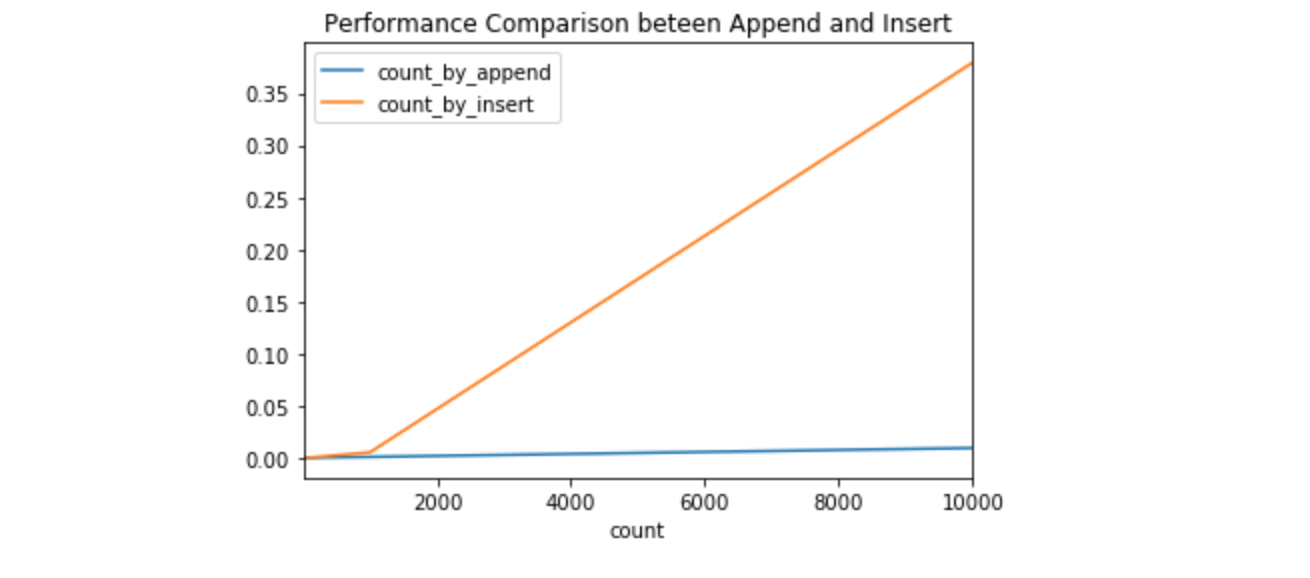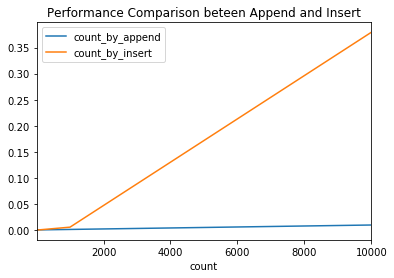# Performance difference between append and insert in Python

## Goal¶

This post aims to compare the performance between append and insert in Python. The performance comparison is simply done by the piece of code that counts a number, append it to a list, and then reverse it.

We will see the significant difference between two codes: one using append is linear and another using insert is quadratic run time growth as below.Reference

## Libraries¶

In :
from timeit import Timer
import pandas as pd
%matplotlib inline


## Append¶

In :
count = 10**5

In :
def count_by_append(count):
nums = []
for i in range(count):
nums.append(i)
nums.reverse()
count_by_append(count)


The execution time is 22ms

## Insert¶

In :
def count_by_insert(count):
nums = []
for i in range(count):
nums.insert(0, i)
count_by_insert(count)


The execution time is 3.53s

## Comparison¶

In :
counts = [10 ** i for i in range(5)]
time_by_append = []
time_by_insert = []

for count in counts:
print(f'Processing {count}')
t = Timer(lambda: count_by_append(count))
time_by_append.append(t.timeit(number=10))
t = Timer(lambda: count_by_insert(count))
time_by_insert.append(t.timeit(number=10))

df_performance = pd.DataFrame({'count': counts,
'count_by_append': time_by_append,
'count_by_insert': time_by_insert})
df_performance

Processing 1
Processing 10
Processing 100
Processing 1000
Processing 10000

Out:
count count_by_append count_by_insert
0 1 0.000013 0.000011
1 10 0.000019 0.000027
2 100 0.000107 0.000227
3 1000 0.000927 0.005318
4 10000 0.009438 0.379457
In :
# Plot the performance difference
df_performance.set_index('count').plot(title='Performance Comparison beteen Append and Insert');